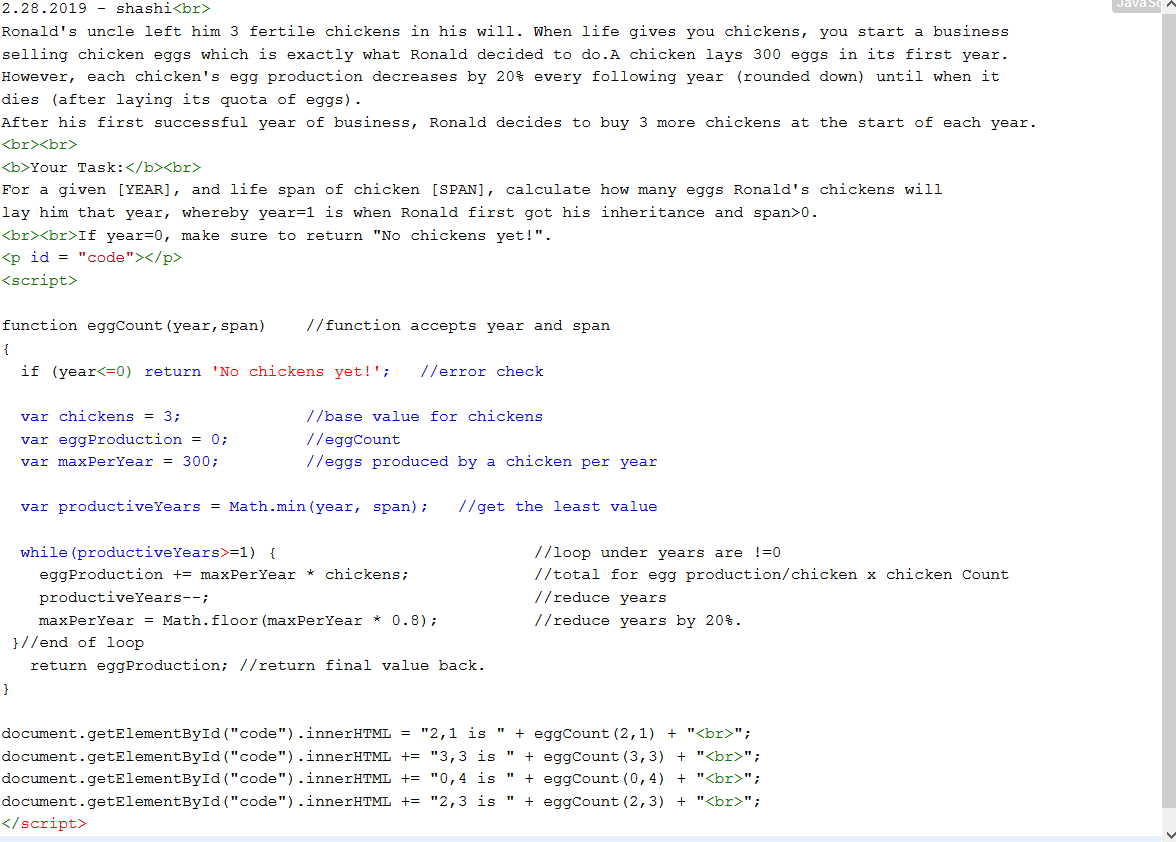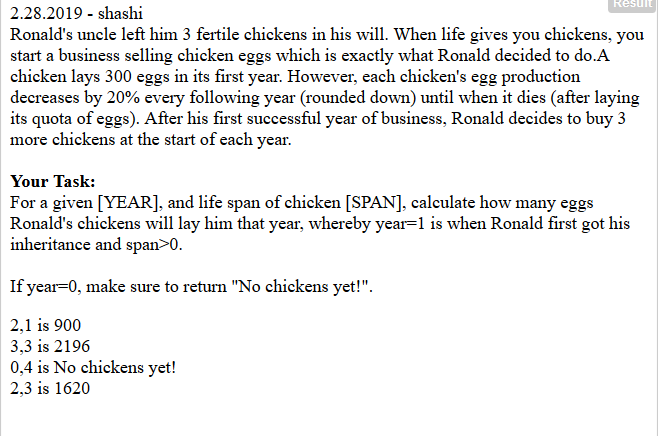2.1.2019 | Print trailing 0s of a factorial

2.2.2019 | Accept 2 numbers and return Binary sum

2.3.2019 | Check for 2 arrays to be balanced

2.4.2019 | Fake Binary number generator

2.5.2019 | Accept array and return count of +ve, sum of -ve numbers

2.6.2019 | Convert to Binary and return count of 1s

2.7.2019 | Accept int array and return formatted phone number

2.8.2019 | Accept array of arrays and return missing array

2.9.2019 | Accept string and return reversed version via recursion

2.10.2019 | Sudoku solver

2.11.2019 | Accept String and return all UPPERCASE positions

2.12.2019 | Mask a 16-digit debit/card number

2.13.2019 | Accept a number and return its reversed version

2.14.2019 | Accept list of elements and reverse the middle part

2.15.2019 | Accept date as String and reduce it to one number by addition

2.16.2019 | Accept numbers as words and return average as word

2.17.2019 | Accept number >1 and generate letter combos based on phone’s dialpad.

2.18.2019 | Accept total amount, coin denominations and return total counting ways

2.19.2019 | Find count of X in an NxN multiplication table

2.20.2019 | Find out if a number is perfect or not.

2.21.2019 | Accept a para of text and return sentence count

2.22.2019 | Wheel of Fortune simulator

2.23.2019 | Accept number and add dashes between the odd digits only.

2.24.2019 | Accept pattern readable by ENIAC Computers and extract readable formats

2.25.2019 | Accept email address and obfuscate it

2.26.2019 | Accept a sentence and shorten it to given length with a connector

2.27.2019 | Accept year and return the century it belongs to

2.28.2019 | Egg production calculator based on years and chicken age span

### 2.1.2019 | Print trailing 0s of a factorial

 #2.1.2019 - Shashi#program to print trailing 0s of a factorial operation.def zeroTrail(number):  #ex: 5 is sent     #Trailing 0 will come into number when any number is multiplied by 10.    zeroCounter = 0  #to track the number of 0s at the end of the final output      while number > 0:  #as long as number > 0        zeroCounter = zeroCounter + number//5  #dividing the number by 5 successfully extracts a 0.        number = number//5 #reduce original number by dividing it by 5.    #end of the loop once number is lesser than 0.        return zeroCounter #out of the loop, return value back to calling function# values <5 do not have factorials ending in 0.print(zeroTrail(5)) #120 - which as 1 0 at the end.print(zeroTrail(10)) #3628800 - which has 2 0s at the end.  print(zeroTrail(12)) #479001600 - which has 2 0s at the end.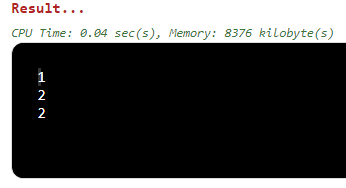### 2.2.2019 | Accept 2 numbers and return Binary sum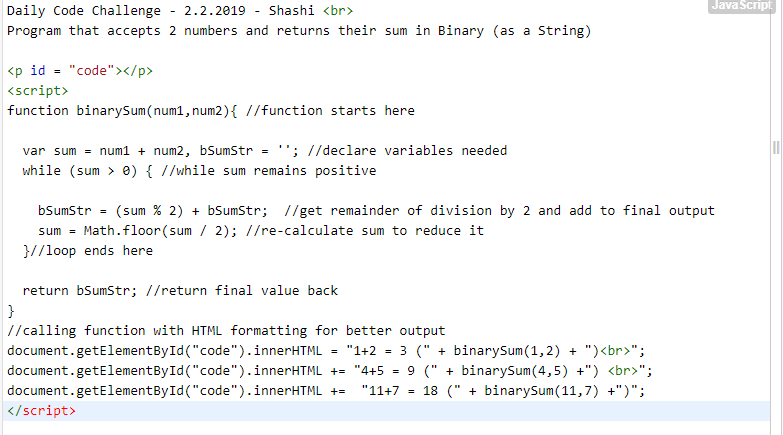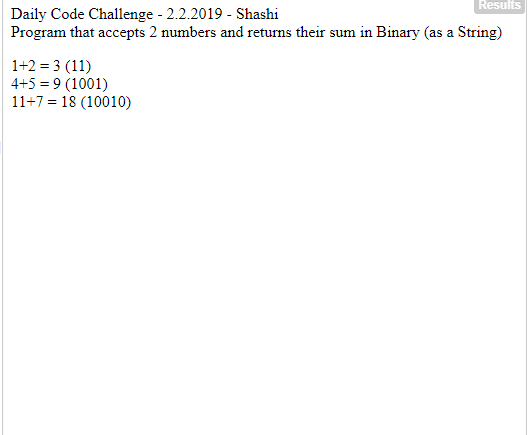### 2.3.2019 | Check for 2 arrays to be balanced

 #2.3.2019 - shashi#program that accepts 2 arrays and checks if they are balanced. as in, contain the same kind of elements.#ex: a,a,a,a,b,b,c  and d,d,d,d,e,e,f# both have similar kind of unique elements appearing same # of times. so these arrays are balanced.def arrayBalanceCheck(array1, array2): #function starts here        #STEP 1: Loading all unique elements from both arrays    set1 = set(array1)  #creating a set loads all the UNIQUE elements only    set2 = set(array2)  #so create such a set of unique elements for both arrays        #STEP 2: Create container arrays to hold the final versions of original arrays    array1_balance = []  #These arrays are empty to begin with but get re-loaded with items    array2_balance = []        for item in set1:  #go through each item in set 1        array1_balance.append(array1.count(item)) #add counts of each element of original array into new balance array        #the purpose of this step is to then compare it with the 2nd array to see if the counts match.    for item in set2: #repeat the loop for all items in set 2        array2_balance.append(array2.count(item)) #add counts of items in original 2nd array into new balance array    if sorted(array1_balance) == sorted(array2_balance):    #if the sorted versions of both arrays match        return "Arrays are balanced."                       #the balance is TRUE    else:                                                   #else        return "Arrays are not balanaced."                  #the balance is FALSE#end of functionarray1 = ["a","b","a","a","b","c"]array2 = ["f","f","f","g","g","h"]print(arrayBalanceCheck(array1, array2))        #elements in both arrays are of same counts.array1 = ["a","a","a","a","b","c"]array2 = ["f","f","g","g","g","h"]print(arrayBalanceCheck(array1, array2))        #elements in both arrays are not of same counts.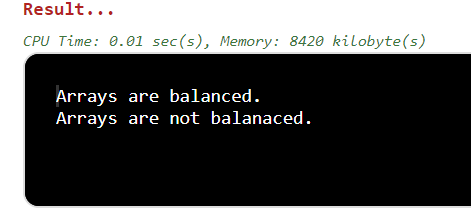### 2.4.2019 | Fake Binary number generator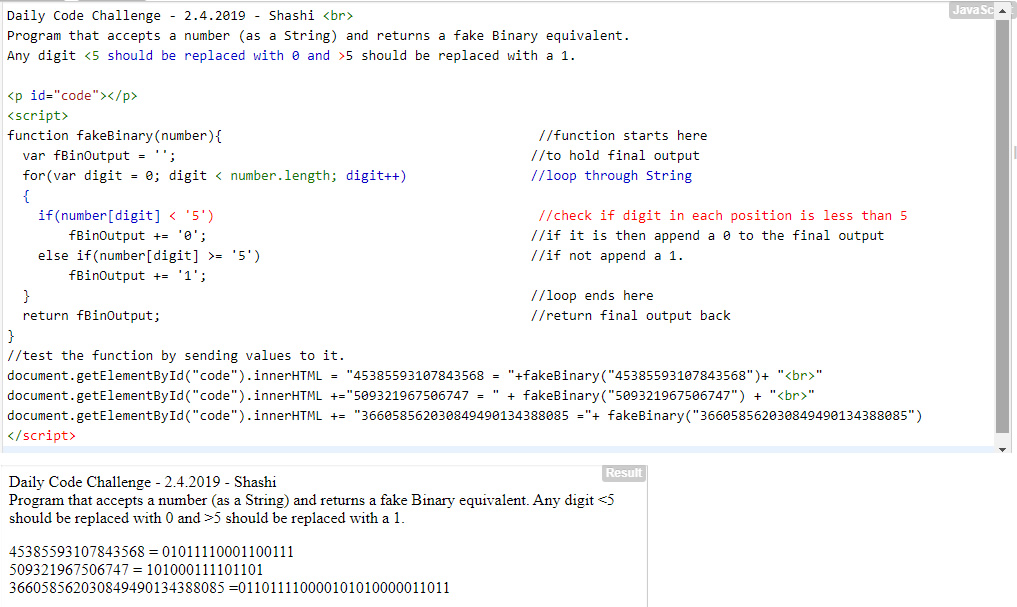### 2.5.2019 | Accept array and return count of +ve, sum of -ve numbers

 //2.5.2019 - Shashi//Program that accepts an integer array vector and returns : Count of positive numbers and Sum of negative numbers//We use Vectors for this solution to help create more flexible, dynamic arrays.using namespace std;#include #include //Function starts herestd::vector countPositivesSumNegatives(std::vector input){    int countPositive=0; //positive counter    int sumNegative=0;   //sum of negative values    if(input.size()==0) return {}; //if input is empty, return blank    //else loop through input array    for(int num=0;num0) countPositive++;     //if value in array >0, add counter      else if(input[num]<0) sumNegative += input[num];  //if value in array <0, create a subtotal    }//loop ends here    return {countPositive,sumNegative}; //return both values to main() or calling function}//end of function//main() starts hereint main(){ //call the function by sending array of values and collect result as vector back std::vector result = countPositivesSumNegatives({0, 2, 3, 0, 5, 6, 7, 8, 9, 10, -1, -2, -3, -4});//loop through vector and print all elements in it for( int i = 0; i < result.size(); i++ )    cout << result[i] << endl;     return 0; //end of main()}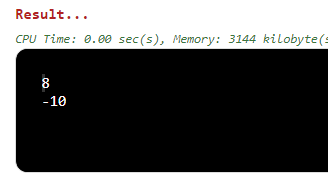### 2.7.2019 | Accept int array and return formatted phone number

 //2.7.2019 - Shashi//C# program that accepts an integer array and returns a String formatted as a phone number.public class formatNumber //class starts here{  public static string createPhoneNumber(int[] numberArray) //function starts here  {      if(numberArray.Length<1 || numberArray.Length>10) //to ensure only 10 digits are processed        return "Invalid Input";      else        return long.Parse(string.Concat(numberArray)).ToString("(000) 000-0000");    //line above does the following from innermost brackets:    //parse and join all the numbers together, conver them to string and format them to "(000) 000-0000" format  }//function ends here     static void Main(string[] args)    {        //Single line that both calls the function and prints the returned output        System.Console.WriteLine(createPhoneNumber(new int[]{1,2,3,4,5,6,7,8,9,0})); //sending an array with the new int[] command        System.Console.WriteLine(createPhoneNumber(new int[]{9,7,4,3,3,1,2,6,7,2,1})); //checking error condition        System.Console.WriteLine(createPhoneNumber(new int[]{9,7,4,3,3,7,2,1})); //values between 1 and 9 get padded with leading 0s        System.Console.WriteLine(createPhoneNumber(new int[]{})); //checking error condition    }//main() ends here}//class ends here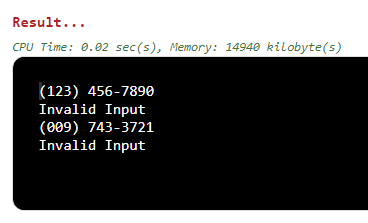### 2.8.2019 | Accept array of arrays and return missing array

 #2.8.2019 - shashi#program that takes an array of arrays. when sorted by length, the size values are consecutive.#program needs to find the missing array in them. #ex: [[1,2], [1,1,2], [2,3,4,5,5]]  should return 4.def findMissingArraySize(masterArray): #function starts here        #STEP 1: first we check for all error possibilities.    if masterArray is None or len(masterArray) == 0: #if master array is empty or contains no elements        return 0  #return 0    else: #then we loop inside the array and check size of EACH array        for array in masterArray:            if array is None or len(array) == 0:  #if any array there is empty                return 0 #return 0    #STEP 2: We load the sizes of all valid arrays into another array    arrayLength = [] #to hold sizes of all valid arrays        for array in masterArray: #loop through each array in master array        arrayLength.append(len(array)) #add the length of each array inside it        #loop ends    arrayLength = sorted(arrayLength) #sort the array by length        #STEP 3: Now we check the array of sizes and see which value is missing (since its sorted)    loop = 0    while loop < len(arrayLength)-1: #loop through the array        if arrayLength[loop+1] - arrayLength[loop] > 1: #check neighboring values. does subtracting them give us more than 1?            return arrayLength[loop] + 1 #if so, then that is the missing array size in the master array. return it.        loop += 1 #else loop on and complete.#running the function with different valuesprint(findMissingArraySize(    [[3, 4], [8, 4, 2, 4], [6,7,8,9,10], ]))     #[2,4,5,1] are the array sizes. so return value is 3print(findMissingArraySize([,[],[2,2]])) #check error conditions. none of the mini-arrays should be blank/none.print(findMissingArraySize(    [['x','x', 'x'], ['x','x','x','x'],['x','x','x','x','x'],['x']])) #[3,4,5,1] are array sizes. so return value is 2.#end of program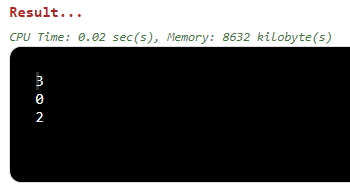### 2.9.2019 | Accept string and return reversed version via recursion

 #2.9.2019 - shashi#program that accepts a string and returns its reversed version via recursion.def revStringRecursive(stringVar): #function starts here                if not stringVar:                       #if empty string sent            return "Invalid"                    #return error message        if len(stringVar) == 1:                 #if only a single letter was sent                return stringVar                    #return the same back        else:                output =  stringVar[-1]             #load the last character into output                      output += revStringRecursive(stringVar[0:len(stringVar)-1]) #append it with a shorter version of string                return output                #if string was "hello world", this function call will take:                                             # 'd' + functionCall("hello worl")                                             # 'dl' + functionaCall("hello wor")                                             # 'dlr' + functionCall("hello wo") ...and so on until complete string is done.                                            #testing the functionprint(revStringRecursive("sample text"))        #valid value testprint(revStringRecursive("12345678"))           #numeric value testprint(revStringRecursive("Hello 1 World 2"))    #mixed type testprint(revStringRecursive("a"))                  #single value testprint(revStringRecursive(""))                   #empty value test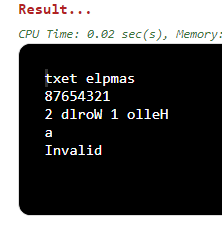### 2.10.2019 | Sudoku solver

 #2.10.2019 - shashi#program that accepts values from a Sudoku board and returns a Boolean#True means the array satisfies Sudoku. False means it does not.solvedPattern = [1, 2, 3, 4, 5, 6, 7, 8, 9]  #array to hold the right values for a solve.def sudokuSolver(sudokuBoard):        #Check columns for the wrong values        for column in sudokuBoard:                      #for every column in array            if sorted(column) != solvedPattern:         #if sorted version of it doesn't match required pattern                return False                            #return false            #Check rows for the wrong values        for row in sudokuBoard:                         #for every row in array            if sorted(row) != solvedPattern:            #if sorted row doesn't match required pattern                return False                            #return false            # check 3x3 grids for presence of 1..9 values        for outerLoop in range(3):                      #loop 3 times outside            for innerLoop in range(3):                  #loop 3 times inside                grid = []                               #array to load up the values in each 3x3 grid into it                    for line in sudokuBoard[outerLoop*3:(outerLoop+1)*3]:                                                   #each iteration loads a different 3x3 grid of the master array                    grid += line[innerLoop*3:(innerLoop+1)*3]                                                  if sorted(grid) != solvedPattern:                               #if such a grid doesn't match required pattern                    return False                                                #return false                                        #for Sudoku board to be solved, each 3x3 grid should contain all numbers from 1..9            #if none of the above falses are returned and code reaches here, then rows/columns and grids are correct.        return True #hence, return true.    #testing out the function by sending a giant array representing the Sudoku board.print(sudokuSolver([         [5, 3, 4, 6, 7, 8, 9, 1, 2],                             [6, 7, 2, 1, 9, 5, 3, 4, 8],                             [1, 9, 8, 3, 4, 2, 5, 6, 7],                             [8, 5, 9, 7, 6, 1, 4, 2, 3],                             [4, 2, 6, 8, 5, 3, 7, 9, 1],                             [7, 1, 3, 9, 2, 4, 8, 5, 6],                             [9, 6, 1, 5, 3, 7, 2, 8, 4],                             [2, 8, 7, 4, 1, 9, 6, 3, 5],                             [3, 4, 5, 2, 8, 6, 1, 7, 9]]))                                                         print(sudokuSolver([         [5, 3, 4, 6, 7, 8, 9, 1, 2],                             [6, 7, 2, 1, 9, 0, 3, 4, 9],                             [1, 0, 0, 3, 4, 2, 5, 6, 0],                             [8, 5, 9, 7, 6, 1, 0, 2, 0],                             [4, 2, 6, 8, 5, 3, 7, 9, 1],                             [7, 1, 3, 9, 2, 4, 8, 5, 6],                             [9, 0, 1, 5, 3, 7, 2, 1, 4],                             [2, 8, 7, 4, 1, 9, 6, 3, 5],                             [3, 0, 0, 4, 8, 1, 1, 7, 9]]))

### 2.11.2019 | Accept String and return all UPPERCASE positions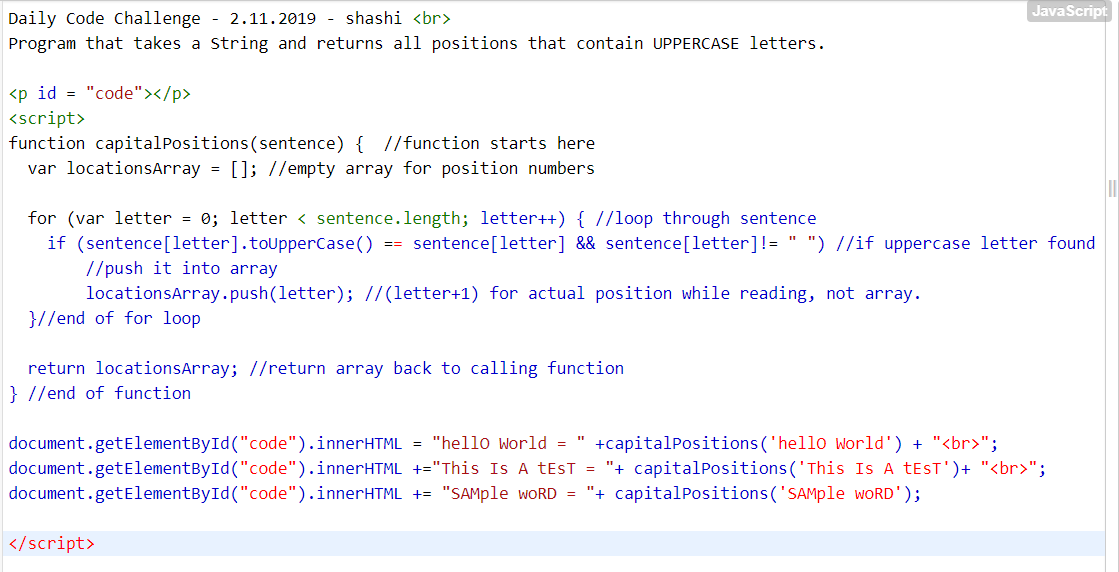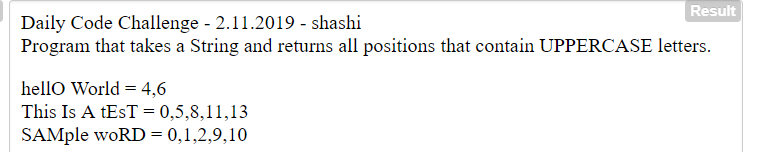### 2.12.2019 | Mask a 16-digit debit/card number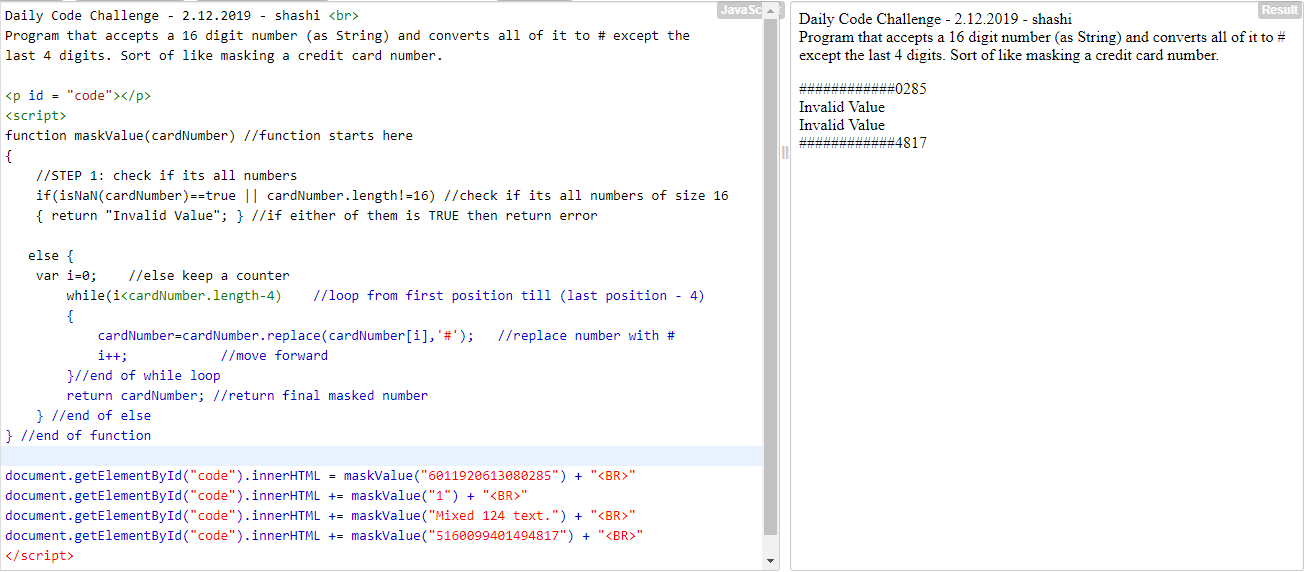### 2.13.2019 | Accept a number and return its reversed version

 #2.13.2019 - shashi#program that takes a number and reverses its digits#ex: 1234 becomes 4321def reverseNumber(number):  #function starts here    if not number:          #erraneous invalid values        return "Invalid"    reverse = int(str(abs(number))[::-1])    #abs(number) > get absolute value of the original number without signs    #str(abs(number)) >  get String/printable version of the absolute number    #int(str(abs(number)) [::-1])  >  reverse all items in the string/printable version and convert it back to int()        #return signed version of number if less than 0. If not then return reverse.    return -reverse if number < 0 else reverse    #testing reverseNumber() with valuesprint(reverseNumber(4321))print(reverseNumber(-117))print(reverseNumber(87))print(reverseNumber(0))#end of program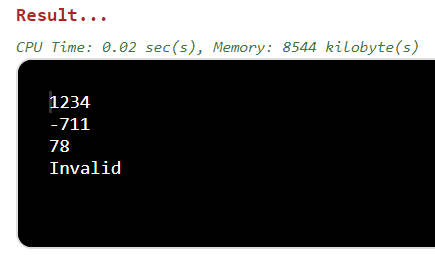### 2.14.2019 | Accept list of elements and reverse the middle part

 #2.14.2019 - shashi#program that accepts a list and returns the middle part of it, reversed.def reverseMiddlePart(myList):                      #function starts here    if not myList:                                  #if empty list sent, return error        return "Invalid Entry"        #else continue here        midPoint = len(myList)//2                      #get the middle point position for the list    if len(myList) % 2 == 0:                       #if the list contains even number of elements     return myList[midPoint-1: midPoint + 1][::-1] # return list[from mid-1 until mid + 1, reversed      else:                                    #if the list contains odd number of elements     return myList[midPoint-1: midPoint + 2][::-1]   return list[mid-1 until mid + 2, reversed (::-1)]    print(reverseMiddlePart([10,11,12,13,14,15,16]))                                        #odd numbersprint(reverseMiddlePart(["quick","brown","fox","jumps","over","the","lazy","dog"]))     #even stringsprint(reverseMiddlePart(['a','e','i','o','u']))                                         #odd charactersprint(reverseMiddlePart([]))                                                            #empty listprint(reverseMiddlePart([10,'a',"mixed",20,'b',"types"]))                         #mixed data types evenprint(reverseMiddlePart([10,'a',"mixed",20,'b']))                                 #mixed data types odd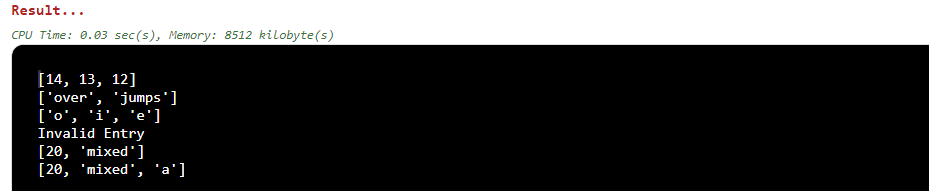### 2.15.2019 | Accept date as String and reduce it to one number by addition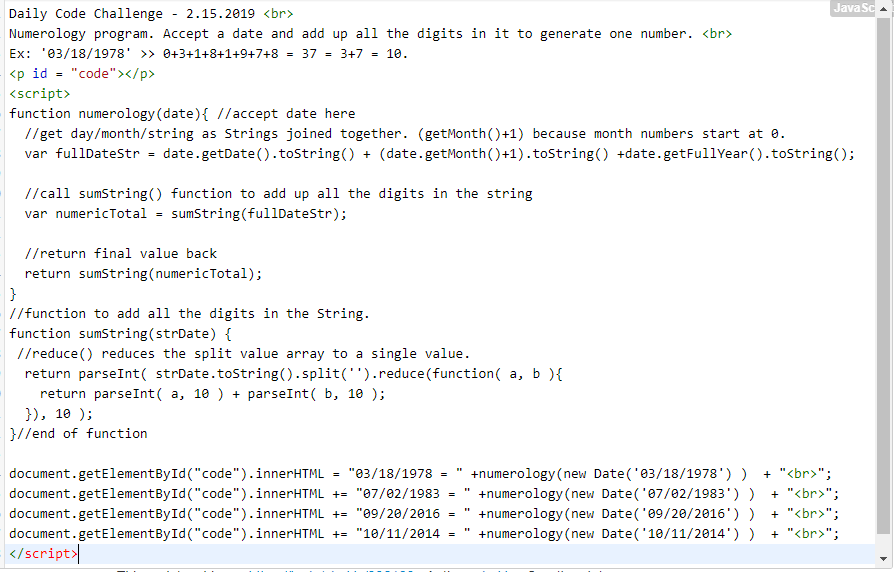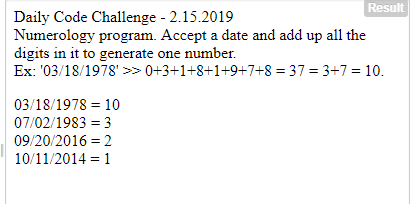### 2.16.2019 | Accept numbers as words and return average as word

 #2.16.2019 - shashi#program accepts a string made up of numbers (spelt out)#it has to then return the average of these numbers back as a string (spelt out)#ex: "one two three four" returns "1+2+3+4 = 10/4 = 2 = two.#only 0-9 are valid inputs.def averageOfStringInts(stringInts):    #function starts here    if not stringInts: return "Invalid"         #invalid/empty value check    #create a lookup array for each of the digits to correspond with a number    valueLookUp = { 'zero'  : 0,            'one'   : 1,            'two'   : 2,            'three' : 3,            'four'  : 4,            'five'  : 5,            'six'   : 6,            'seven' : 7,            'eight' : 8,            'nine'  : 9 }        #variable to hold int total            total = 0    #split the original string into an array[]            stringInts = stringInts.split(' ')    for number in range(len(stringInts)):            #loop through the array        if stringInts[number] in valueLookUp:        #if string number found in lookup array            total += valueLookUp[stringInts[number]] #get the int equivalent and add to result        else:            return "N/A"                             #if not found return error.                averageResult = total // len(stringInts)          #now get the average value as integer.    for number in valueLookUp:                          #finally look up word equivalent of average number            if valueLookUp.get(number) == averageResult:    #if value found in lookup array, then return it.            averageResult = number    return averageResult#end of functionprint(averageOfStringInts("one two three"))    #1+2+3+4 = 10/4 = 2 = twoprint(averageOfStringInts("six one eight"))         #6+1+8 = 15/3 = 5 = fiveprint(averageOfStringInts(""))                      #error check - invalidprint(averageOfStringInts("nineteen eight five"))   #missing value check - N/A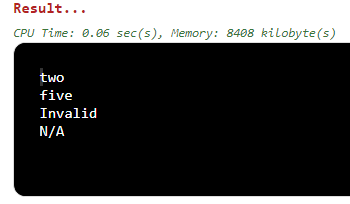### 2.17.2019 | Accept number >1 and generate letter combos based on phone’s dialpad.

 #2.17.2019 - shashi#program that accepts a number and prints out all possible word combos as represented on a phone dialpad.#So: 2 = "abc", 3 = "def", 4 = "ghi"....etc until 9.#If 235 is sent, then "abc","def" and "jkl" are combined to generate word combos.def wordCombosDialpad(number):        #error check:    if not number: return "Invalid"        #step 1: create lookup for each of the digits.    dialPadLookUp = {'2': 'abc','3': 'def','4': 'ghi','5': 'jkl','6': 'mno','7': 'pqrs','8': 'tuv','9': 'wxyz',}      #step 2: convert int input to string and set up output array    strNumber = str(number)    comboArray = [''] #empty array to hold all combos    #step 3 : loop through each letter of converted string and lookup its combo from lookup array    for char in strNumber:  #loop through each character in strNumber        letters = dialPadLookUp.get(char, '')   #look up its mapped combo string in dialPadLookUp                #something new I learnt. Combining loops within the array value assignment to continously loop through each pattern and join with the other.        #so, "abc" and "def" would go: "ad", "ae", "af", "bd","be","bf" "cd", "ce", "cf"        comboArray = [value+eachLetter for value in comboArray for eachLetter in letters]    #loop ends here        #return final array back    return comboArray print(wordCombosDialpad(23))        #take mapping of 2 and 3 and combine them. 9 combinationsprint(wordCombosDialpad(764))       #mapping of 7 (pqrs), 6 (mno) and 4 (ghi) together.  36 combinationsprint(wordCombosDialpad(8324))      #mapping of 8 (tuv), 3 (def), 2(abc), 4(ghi) together. 81 combosprint(wordCombosDialpad(0))         #error handling. Invalid returned.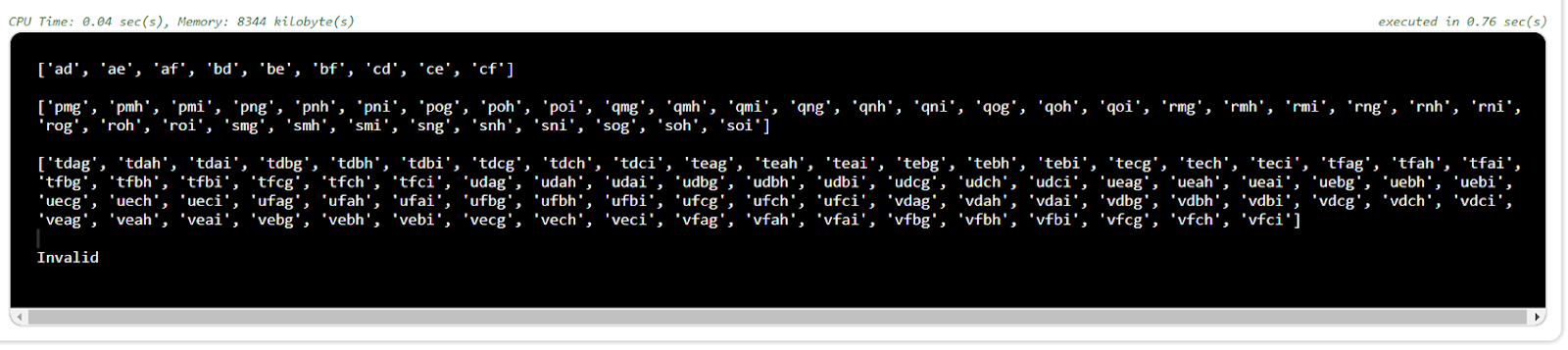### 2.18.2019 | Accept total amount, coin denominations and return total counting ways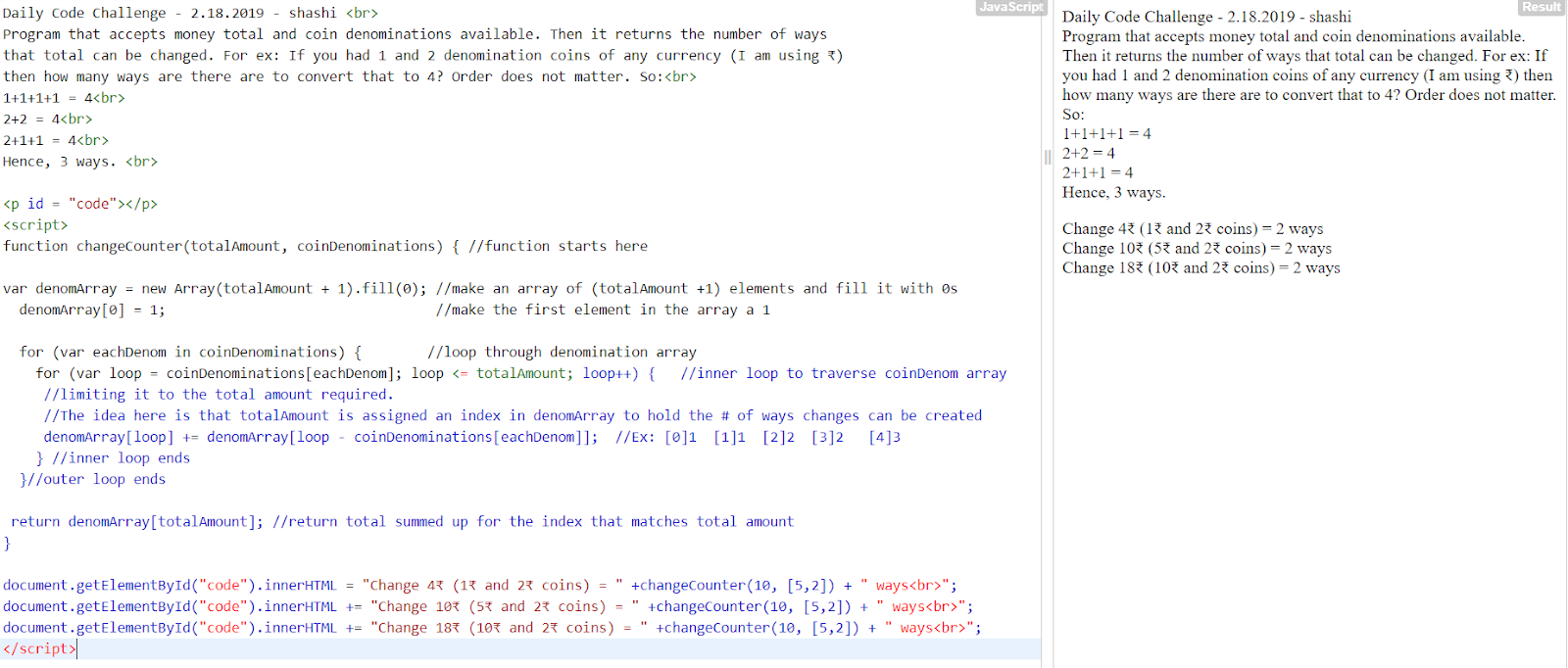### 2.19.2019 | Find count of X in an NxN multiplication table

 #2.19.2019 - shashi#program that accepts N and X and returns the number of times X appears in an NxN multiplication table.#for ex: N=4, X= 6.#1 2 3#2 4 6#3 6 9#4 8 12#so, 6 appears 2 times in the multiplication table.def getCountMultiply(sourceNumber, finderValue):    if sourceNumber == 1:           #checking for 1        return sourceNumber            count =0        #count of occurrences    #STEP 1: Generating the NxN table and populating it. Shorter version possible but expanding it here.    table = [[0 for x in range(sourceNumber)] for y in range(sourceNumber)]    for row in range(sourceNumber):        for col in range(sourceNumber):            table[row][col]=(row+1)*(col+1)        #STEP 2: Going through NxN table and looking for value needed    for row in range(sourceNumber):        for col in range(sourceNumber):            if(table[row][col] == finderValue): #if value found                count = count+1                 #increase counter hit        return count  #return final count back to calling function#testing functionprint(getCountMultiply(4,6))    #2 6sprint(getCountMultiply(5,14))    #0 14sprint(getCountMultiply(10,20))    #4 20sprint(getCountMultiply(1,5))    #1 sent. so 1 returns regardless of second parameter.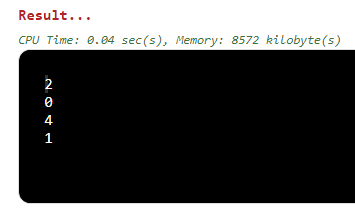### 2.20.2019 | Find out if a number is perfect or not.

 #2.20.2019 - shashi#program accepts a number and returns whether or not it is a perfect number.#perfect number is one whose proper divisors, when added up, equal to the number (excluding itself).#ex: 6. 1, 2 and 3 divide it perfectly. So 1+2+3 = 6.#ex: 28. 1,2,4,7 and 14 divide it perfectly. So, 1+2+4+7+14 = 28.#Complete list of perfect numbers >> https://en.m.wikipedia.org/wiki/List_of_perfect_numbersdef perfectCheck(number):   #function starts here                 if(number <1): return "Invalid"        #STEP 1: Variable to hold sum of proper divisors of original number        numberSum = 1                  #STEP 2: Look for proper divisors and add them together (except the number itself)            looperValue = 2                                       #imagine number = 6        while looperValue * looperValue < number:              #loop until looperValue^2 < number                    if number % looperValue == 0:                         #if looperValue divides number properly                 numberSum = numberSum + looperValue                  #add that value to numberSum       #add quotient of division. We have to make numberSum equal to original number.             numberSum = numberSum + (number/looperValue)                     looperValue += 1                                         #loop progress                    #STEP 3: if the sum of proper divisors of number matches number, then number is PERFECT.        if(numberSum ==number and number!=1):           #if divisor sum equals to number and number is not 1            return True                                 #it is a perfect number        else:                                           #else it is not.            return False#end of functionprint(perfectCheck(6))              #trueprint(perfectCheck(24))             #falseprint(perfectCheck(28))             #trueprint(perfectCheck(-1))             #error check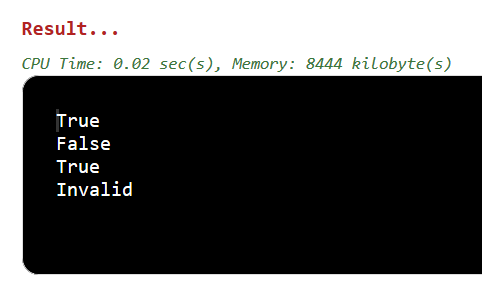### 2.21.2019 | Accept a para of text and return sentence count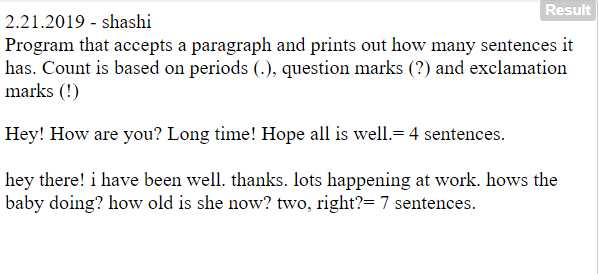### 2.22.2019 | Wheel of Fortune simulator

 #2.22.2019 - shashi#wheel of fortune simulator.#input sent is contestname and scores. winner is one who is closest to 100 (while still being lower or equal to 100). def WOF_Winner(playerData): #get array data        winnerName= ""          #to hold name of winner    winnerScore = 0             playerTotal = 0        #loop through each player data in the object array    for eachPlayer in playerData:                          playerName = eachPlayer['name']             #load player name        playerScore = eachPlayer['scores']          #load player score                    totalScore = 0                         spinCount = 0                playerTotal += 1                #number of players                for eachScore in playerScore:       #nested loop to track each score entry for each player            if eachScore > 100 or eachScore % 5:        #if invalid value in scores                return False                            #no point going on. so return false.                            totalScore += eachScore                     #else increment totalScore for this player.            spinCount += 1                              #increment spins he/she got.                    if spinCount not in (1, 2):                     #invalid spin counts (only one or two allowed)                    return False                                #return false        elif totalScore > winnerScore:                  #get highest winner's score and name            winnerScore = totalScore            winnerName = playerName    #end of for loop here    #check if score is within 100 and there were 3 players    if winnerScore<=100 and playerTotal ==3:        return winnerName                   #get the winner's name    else:        return False#testing function trial 1player1 = {"name": "Alice", "scores": [10, 45,5]}player2 = {"name": "Bob", "scores": [70,5]}player3 = {"name": "Cathy", "scores": [35, 55]}print(WOF_Winner([player1, player2, player3])) #Returns False because Alice got 3 spins. Not allowed.#testing function trial 2player1 = {"name": "John", "scores": [20, 40]}player2 = {"name": "Jane", "scores": [80,15]}player3 = {"name": "Erik", "scores": }      #this is OK since 1 or 2 spins allowed.print(WOF_Winner([player1, player2, player3])) #Returns Jane since she is closer to 100.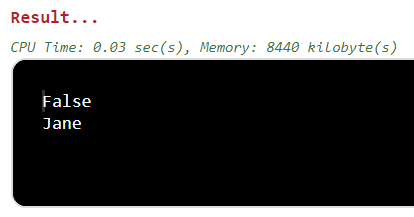### 2.23.2019 | Accept number and add dashes between the odd digits only.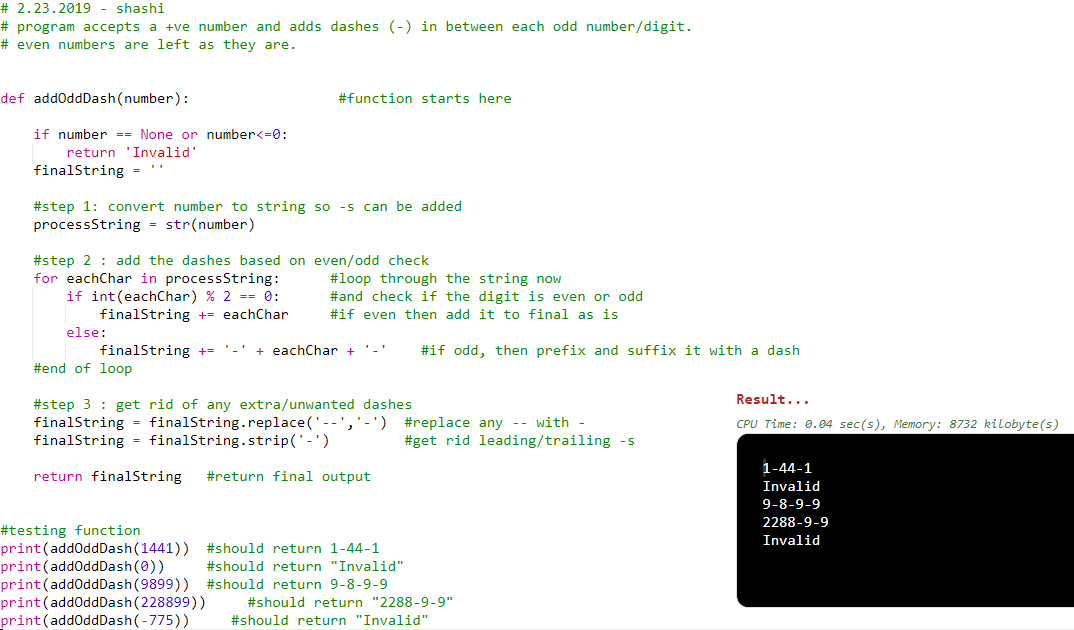### 2.24.2019 | Accept pattern readable by ENIAC Computers and extract readable formats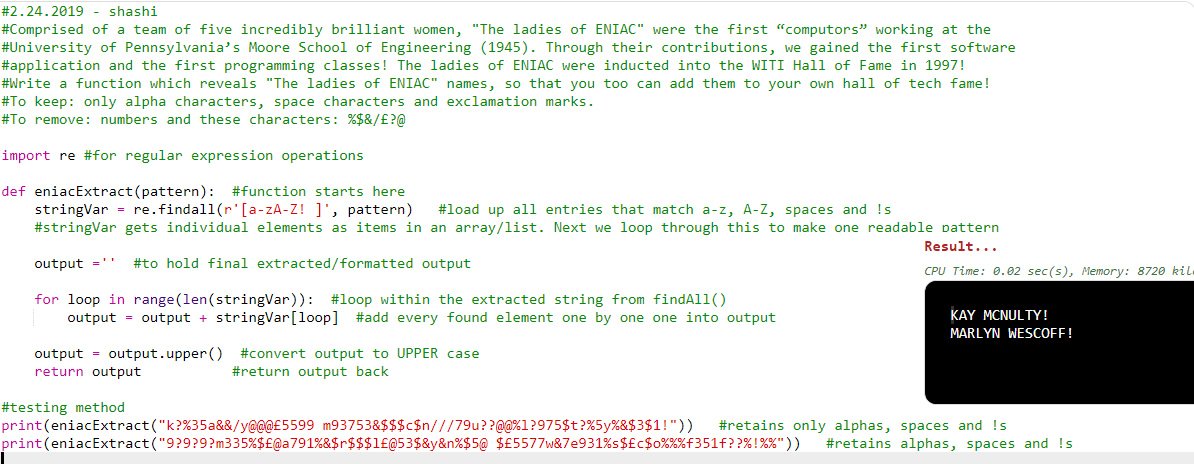### 2.25.2019 | Accept email address and obfuscate it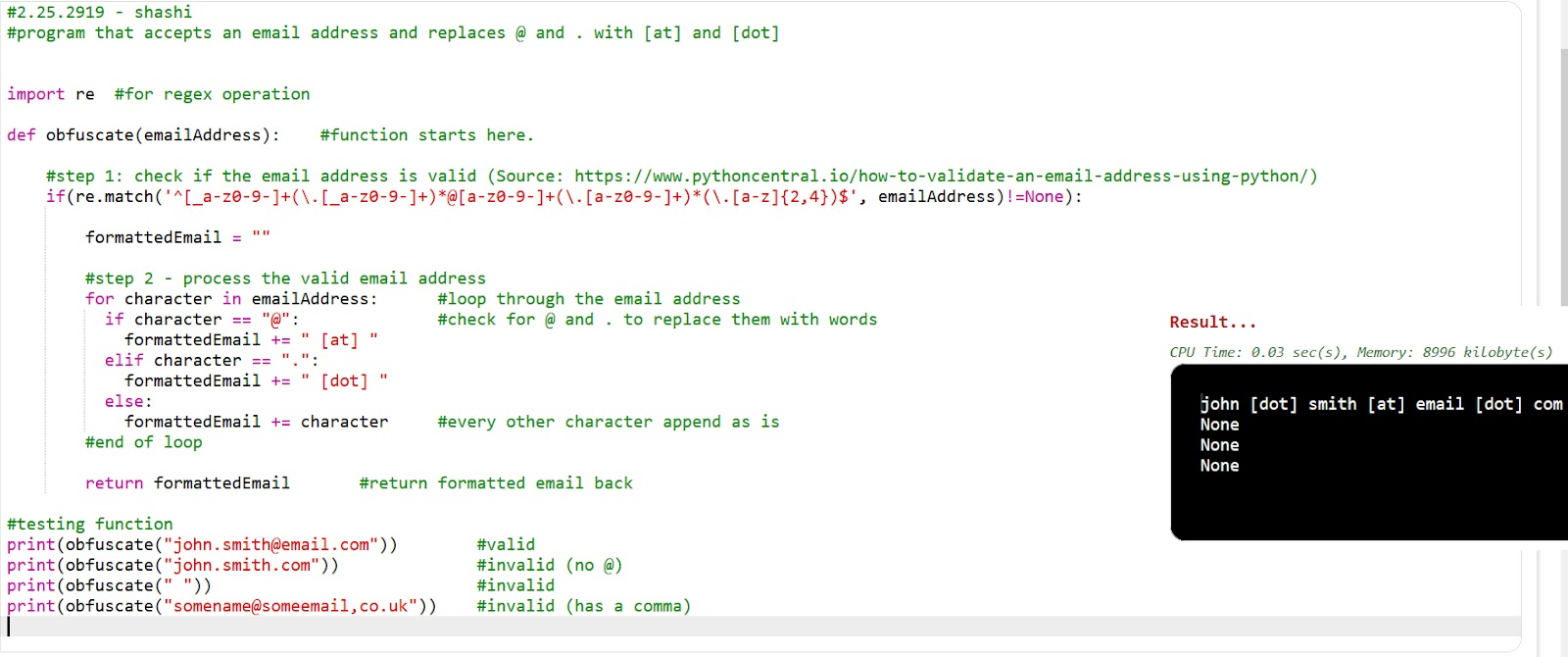### 2.26.2019 | Accept a sentence and shorten it to given length with a connector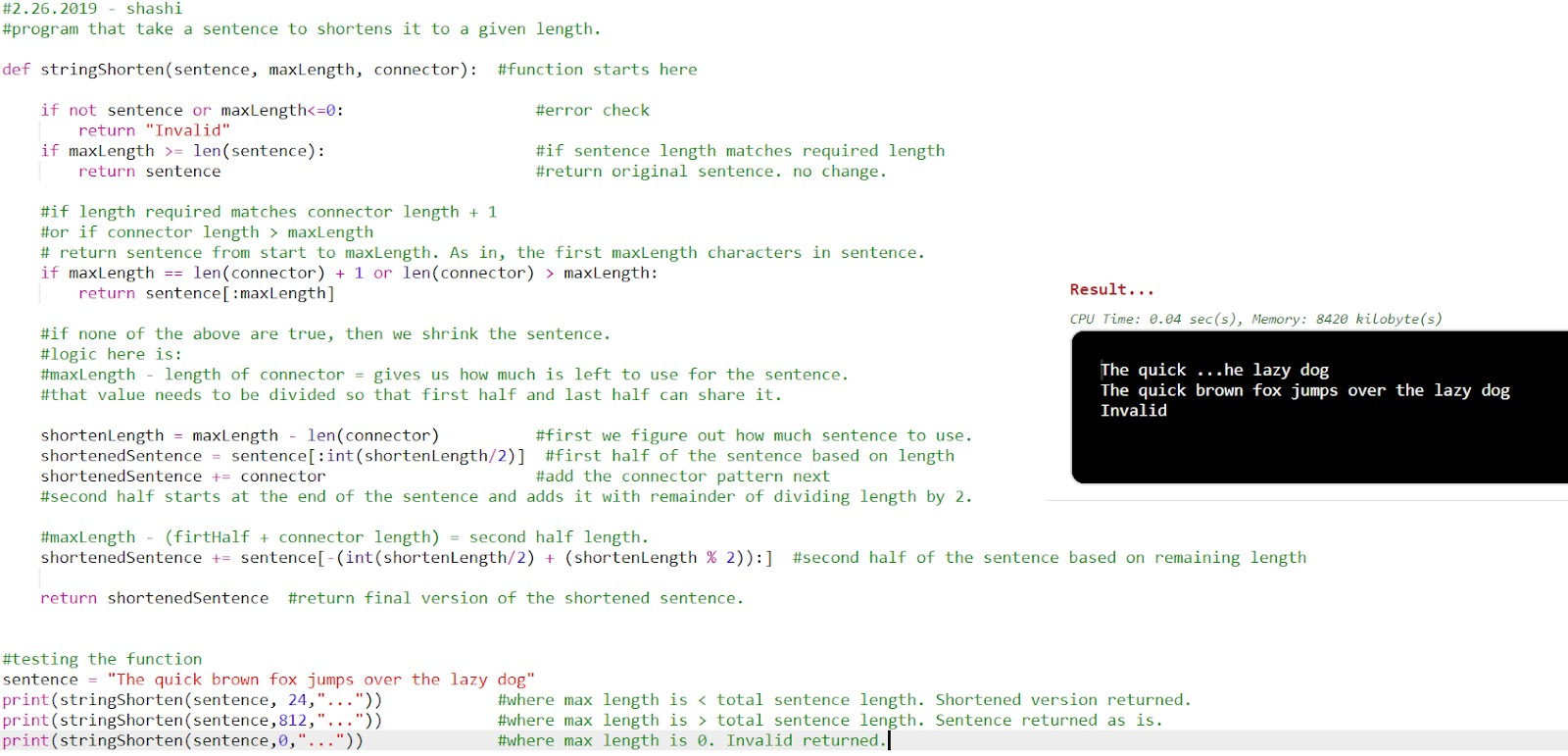### 2.27.2019 | Accept year and return the century it belongs to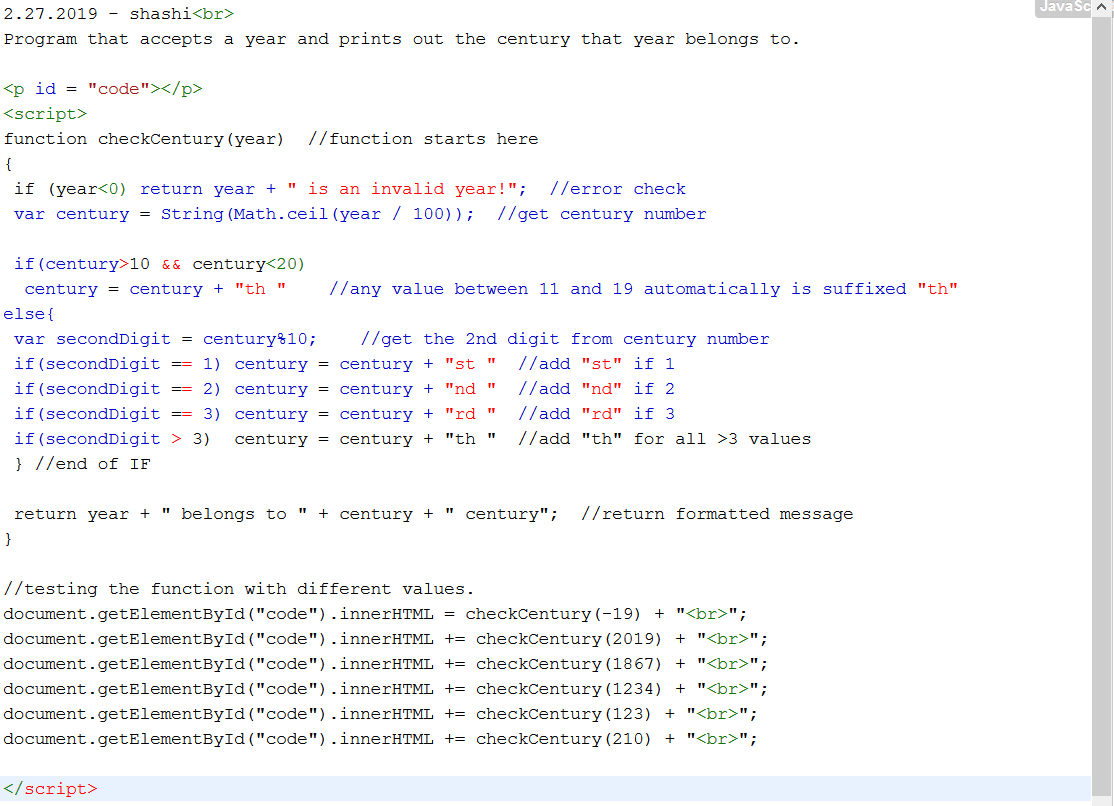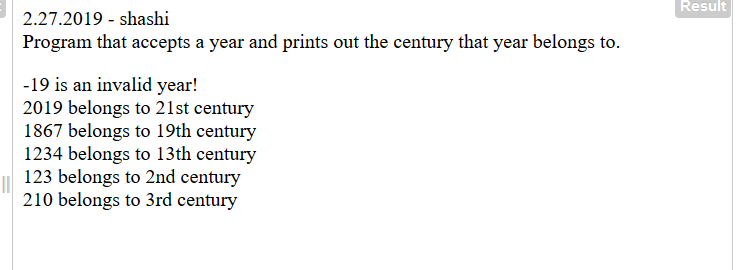### 2.28.2019 | Egg production calculator based on years and chicken age span